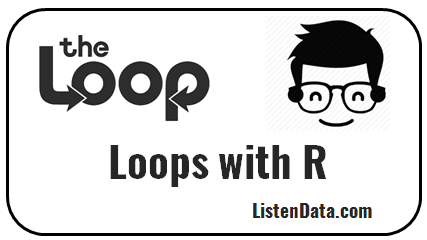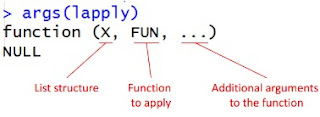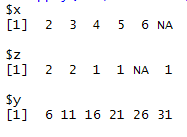# Loop in R

This tutorial explains how to write loop in R. It includes explanation of APPLY family of functions and FOR LOOP with several examples which makes writing R loops easy.Loops with R
What is Loop?

Loop helps you to repeat the similar operation on different variables or on different columns or on different datasets. For example, you want to multiple each variable by 5. Instead of multiply each variable one by one, you can perform this task in loop. Its main benefit is to bring down the duplication in your code which helps to make changes later in the code.

Ways to Write Loop in R
1. For Loop
2. While Loop
3. Apply Family of Functions such as Apply, Lapply, Sapply etc

Apply Family of Functions

They are the hidden loops in R. They make loops easier to read and write. But these concepts are very new to the programming world as compared to For Loop and While Loop.

1. Apply Function

It is used when we want to apply a function to the rows or columns of a matrix or data frame. It cannot be applied on lists or vectors.apply arguments

Create a sample data set
dat <- data.frame(x = c(1:5,NA),
z = c(1, 1, 0, 0, NA,0),
y = 5*c(1:6))

Example 1 : Find Maximum value of each row
apply(dat, 1, max, na.rm= TRUE)
Output :  5 10  15  20 25 30

In the second parameter of apply function, 1 denotes the function to be applied at row level.

Example 2 : Find Maximum value of each column
apply(dat, 2, max, na.rm= TRUE)
The output is shown in the table below -
x z y
5 1 30
In the second parameter of apply function, 2 denotes the function to be applied at column level.

2. Lapply Function

When we apply a function to each element of a data structure and it returns a list.lapply arguments

Example 1 : Calculate Median of each of the variables
lapply(dat, function(x) median(x, na.rm = TRUE))
The function(x) is used to define the function we want to apply. The na.rm=TRUE is used to ignore missing values and median would now be calculated on non-missing values.

Example 2 : Apply a custom function
lapply(dat, function(x) x + 1)
In this case, we are adding 1 to each variables and the final output would be a list and output is shown in the image below.Output

3. Sapply Function

Sapply is a user friendly version of Lapply as it returns a vector when we apply a function to each element of a data structure.

Example 1 : Number of Missing Values in each Variable
sapply(dat, function(x) sum(is.na(x)))
The above function returns 1,1,0 for variables x,z,y in data frame 'dat'.

Example 2 : Extract names of all numeric variables in IRIS dataset
colnames(iris)[which(sapply(iris,is.numeric))]
In this example, sapply(iris,is.numeric) returns TRUE/FALSE against each variable. If the variable is numeric, it would return TRUE otherwise FALSE. Later,  which function returns the column position of the numeric variables . Try running only this portion of the code which(sapply(iris,is.numeric)). Adding colnames function would help to return the actual names of the numeric variables.

Lapply and Sapply Together

In this example, we would show you how both lapply and sapply are used simultaneously to solve the problem.

Create a sample data
dat <- data.frame(x = c(1:5,NA),
z = c(1, 1, 0, 0, NA,0),
y = factor(5*c(1:6)))

Converting Factor Variables to Numeric

The following code would convert all the factor variables of data frame 'dat' to numeric types variables.
index <- sapply(dat, is.factor)
dat[index] <- lapply(dat[index], function(x) as.numeric(as.character(x)))
Explanation :
1. index would return TRUE / FALSE whether the variable is factor or not
2. Converting only those variables wherein index=TRUE.

4. For Loop

Like apply family of functions, For Loop is used to repeat the same task on multiple data elements or datasets. It is similar to FOR LOOP in other languages such as VB, python etc. This concept is not new and it has been in the programming field over many years.

Example 1 : Maximum value of each column
x = NULL
for (i in 1:ncol(dat)){
x[i]= max(dat[i], na.rm = TRUE)}
x
Prior to starting a loop, we need to make sure we create an empty vector. The empty vector is defined by x=NULL. Next step is to define the number of columns for which loop over would be executed. It is done with ncol function. The length function could also be used to know the number of column.

The above FOR LOOP program can be written like the code below -
x = vector("double", ncol(dat))
for (i in seq_along(dat)){
x[i]= max(dat[i], na.rm = TRUE)}
The vector function can be used to create an empty vector. The seq_along finds out what to loop over.

Example 2 : Split IRIS data based on unique values in "species" variable

The program below creates multiple data frames based on the number of unique values in variable Species in IRIS dataset.
for (i in 1:length(unique(iris\$Species))) {
require(dplyr)
assign(paste("iris",i, sep = "."), filter(iris, Species == as.character(unique(iris\$Species)[i])))
}
It returns three data frames named iris.1 iris.2 iris.3.

Combine / Append Data within LOOP

In the example below, we are combining / appending rows in iterative process. It is same as PROC APPEND in SAS.

Method 1 : Use do.call with rbind

do.call() applies a given function to the list as a whole. When it is used with rbind, it would bind all the list arguments. In other words, it converts list to matrix of multiple rows.
temp =list()
for (i in 1:length(unique(iris\$Species))) {
series= data.frame(Species =as.character(unique(iris\$Species))[i])
temp[[i]] =series
}
output = do.call(rbind, temp)
output
Method 2 :  Use Standard Looping Technique

In this case, we are first creating an empty table (data frame). Later we are appending data to empty data frame.
dummydt=data.frame(matrix(ncol=0,nrow=0))
for (i in 1:length(unique(iris\$Species))) {
series= data.frame(Species =as.character(unique(iris\$Species))[i])
if (i==1) {output = rbind(dummydt,series)}  else {output = rbind(output,series)}
}
output
If we need to wrap the above code in function, we need to make some changes in the code. For example, data\$variable won't work inside the code . Instead we should use data[[variable]]. See the code below -
dummydt=data.frame(matrix(ncol=0,nrow=0))
temp = function(data, var) {
for (i in 1:length(unique(data[[var]]))) {
series= data.frame(Species = as.character(unique(data[[var]]))[i])
if (i==1) {output = rbind(dummydt,series)}  else {output = rbind(output,series)}
}
return(output)}
temp(iris, "Species")

For Loop and Sapply Together

Suppose you are asked to impute Missing Values with Median in each of the numeric variable in a data frame. It's become a daunting task if you don't know how to write a loop. Otherwise, it's a straightforward task.

In the program below, which(sapply(dat, is.numeric)) makes sure loop runs only on numeric variables.
for (i in which(sapply(dat, is.numeric))) {
dat[is.na(dat[, i]), i] <- median(dat[, i],  na.rm = TRUE)
}

Create new columns in Loop

Suppose you need to standardise multiple variables. To accomplish this task, we need to execute the following steps -
1.  Identify numeric variables
2. Calculate Z-score i.e. subtracting mean from original values and then divide it by standard deviation of the raw variable.
3. Run Step2 for all the numeric variables
4. Make names of variables based on original names. For example x1_scaled.

Create dummy data
mydata = data.frame(x1=sample(1:100,100), x2=sample(letters,100, replace=TRUE), x3=rnorm(100))
Standardize Variables
lst=list()
for (i in which(sapply(mydata, is.numeric))) {
x.scaled = (mydata[,i] - mean(mydata[,i])) /sd(mydata[,i])
lst[[i]] = x.scaled
}

names(lst) <- paste(names(sapply(mydata, is.numeric)),"_scaled", sep="")
mydata.scaled= data.frame(do.call(cbind, lst))
In this case, do.call with cbind function helps to make data in matrix form from list.

5. While Loop in R

A while loop is more broader than a for loop because you can rescript any for loop as a while loop but not vice-versa.

In the example below, we are checking whether a number is an odd or even,
i=1
while(i<7)
{
if(i%%2==0)
print(paste(i, "is an Even number"))
else if(i%%2>0)
print(paste(i, "is an Odd number"))
i=i+1
}
The double percent sign (%%) indicates mod. Read i%%2 as mod(i,2). The iteration would start from 1 to 6 (i.e. i<7). It stops when condition is met.

Output:
 "1 is an Odd number"
 "2 is an Even number"
 "3 is an Odd number"
 "4 is an Even number"
 "5 is an Odd number"
 "6 is an Even number"

Loop Concepts : Break and Next

Break Keyword

When a loop encounters 'break' it stops the iteration and breaks out of loop.
for (i in 1:3) {
for (j in 3:1) {
if ((i+j) > 4) {
break    } else {
print(paste("i=", i, "j=", j))
}
}
}
Output :
 "i= 1 j= 3"
 "i= 1 j= 2"
 "i= 1 j= 1"

In this case, as condition i+j >4 is met, it breaks out of loop.

Next Keyword

When a loop encounters 'next', it terminates the current iteration and moves to next iteration.
for (i in 1:3) {
for (j in 3:1) {
if ((i+j) > 4) {
next
} else {
print(paste("i=", i, "j=", j))
}
}
}

Output :
 "i= 1 j= 3"
 "i= 1 j= 2"
 "i= 1 j= 1"
 "i= 2 j= 2"
 "i= 2 j= 1"
 "i= 3 j= 1"

If you get confused between 'break' and 'next', compare the output of both and see the difference.Share
Related PostsDeepanshu founded ListenData with a simple objective - Make analytics easy to understand and follow. He has over 10 years of experience in data science. During his tenure, he has worked with global clients in various domains like Banking, Insurance, Private Equity, Telecom and Human Resource.

1 Response to "Loop in R"
1.I did not understand this-
For example, data\$variable won't work inside the code . Instead we should use data[[variable]].

data\$Species when I am accessing inside function is working.

Next → ← Prev
Love this post? Support Us!